1 – Intro To Matrix Math (Matrix Algebra Tutor) – Learn how to Calculate with Matrices1 – Intro To Matrix Math (Matrix Algebra Tutor) – Learn how to Calculate with Matrices

This is just a few minutes of a complete course.
Get full lessons & more subjects at: http://www.MathTutorDVD.com.

In this lesson, the student will learn what a matrix is in algebra and how to perform basic operations on matrices. We will learn how to do basic matrix math operations by solving example problems.

algebra tutors,matrices,matrix math,matrix algebra,add matrices,calculate,linear,matrix lesson,matrices lesson,algebra of matrices,matrix math calculator,matrix math problems,matrix math rules,matrix math example,matrix algebra rules,matrix algebra definition,add two matrices,what is a matrix,what are matrices,algebra,algebra 2,college algebra,linear algebra,calculate with matrices,matrix calculation

algebratutors.org

25 Responses to “1 – Intro To Matrix Math (Matrix Algebra Tutor) – Learn how to Calculate with Matrices”

1.Janith Jeewantha says:

sir can we solve concurrent equations with matrix

2.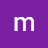madaxey cali says:

If ever was there a natural born teacher, this fellow must be it! Thank you, sir.

3.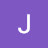Jacob Matura says:

good work. i need more examples…..

4.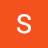Simon Titus says:

Great teacher will explanation

5.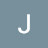brofallo says:

This guy is so comforting

6.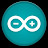Surekha Ghorpade says:

l am truly beginner🤯 but sir your are best🌟

7.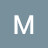hyped soul says:

Thank you so much for the lecture… Straight to my question,Is the element arrangement important to consider while considering equality of matrices…lets A23 = 4 , B23= 5 yet all the single elements are found in both matrices ,the difference is the arrangement

8.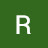Rajender Kashyap says:

Nice

9.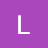7813339920 Lacombe says:

Wow! Thank you so much may God bless you.

10.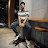Ahmed Sokkar says:

watching in 2021

11.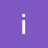i am the one says:

12.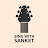Learn with Sanket says:

My teacher to me: Explain Matrix!!
Me: (gives this video)
😝🤣

13.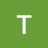Tushar Shorma says:

Rate my professor 5/5

14.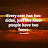Wahid Darwish says:

sir, you are awesome!

15.7813339920 Lacombe says:

Wow! Thank you so much.

16.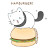Akatsuki Hajime says:

Basically, its algebra but is a big scale.

17.Akatsuki Hajime says:

Good to watch when couldn't sleep at night

I am just joking

18.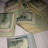johnesha harris says:

This my first time watching I think I'm going to use wen I'm the military

19.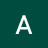Alice Musara says:

It's useful ,l really like your teachings

20.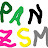Eva Dugina says:

great teacher for students who have difficulties with math… really very easy and really sloooowww…….. like!

21.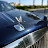sg gamer says:

thanks alot

22.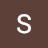Swadesh Taneja says:

Very simple

23.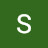Sohail Janjua says:

24.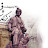Amir Nazari says:
25.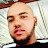Natto_XD says: